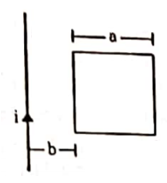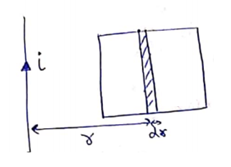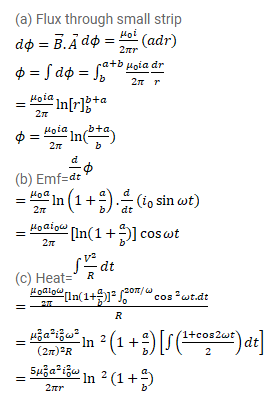# Figure shows a square frame of wire having a total resistance placed coplanarly with a long,

Question:

Figure shows a square frame of wire having a total resistance placed coplanarly with a long, straight wire. The wire carries a current i given by $i=i_{0} \sin \omega t$. Find

(a) the flux of the magnetic field through the square frame,

(b) the emf induced in the frame and

(c) the heat developed in the frame in the time interval 0 to $\frac{20 \pi}{\omega}$.Solution: